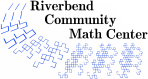# Demystifying Division

### Description

Students develop an understanding of division of whole numbers using sharing and grouping models to solve problems.

1 hour

8 lessons

### Topics

• whole number division
• relationships between division, multiplication, subtraction, and addition
• division notation
• meaning of remainders
• problem solving
• communicating and modeling mathematical ideas

### Goals

• Students will be able to model division problems with partitioning (sharing) and grouping (repeated addition).
• Students will be able to write division equations using two symbols: the standard division symbol and fractions.
• Students will be able to correctly interpret remainders within the context of a given problem.
• Students will be able to use multiplication, subtraction, and addition equations which express ideas equivalent to a given division equation.
• Students will be introduced to long division using a partial quotients strategy.
• Students will be able to communicate their mathematical thinking verbally, with models or pictures, and in writing.

### Materials

• "The Doorbell Rang" by Pat Hutchins
• "One Hundred Hungry" Ants by Elinor J Pinczes
• a large quantity of pennies (approx. 300)
• chart paper
• 24 tiles or other tokens per student
• small cup or bowl
• 5" x 8" cards
• activity sheets - "Activity 1" - "Activity 2" - "How Many Stars?"
• cookie cereal for first lesson (optional)
• small white boards and dry erase markers (optional)
• post-its (optional)

### Prerequisites

• Students are able to count by tens.
• Students have worked with multiplication models.

### Credits

• 1. Marilyn Burns, Lessons for Introducing Division: Grades 3-4
• 2. Van de Walle and Lovin, Teaching Student-Centered Mathematics Grades 5-8, Vol. 3

### Authors

Cheryl A. Nix, M.S.

### National Common Core Standards

 3.OA.2 Interpret whole-number quotients as objects partitioned into equal shares. 3.OA.3 Use multiplication and division within 100 to solve word problems in situations involving equal groups, arrays, and measurements quantities. 3.OA.4 Determine the unknown whole number in a multiplication or division equation relating three whole numbers. 4.OA.3 Solve multistep word problems posed with whole numbers and having whole-number answers using the four operations, including problems in which remainders must be interpreted.
Riverbend Community Math Center
hello@riverbendmath.org
http://riverbendmath.org
(574) 339-9111This work placed into the public domain by the Riverbend Community Math Center.